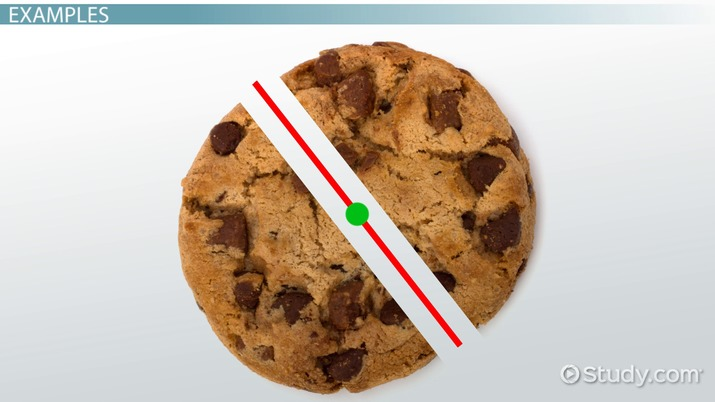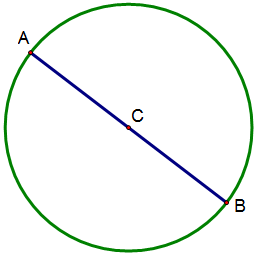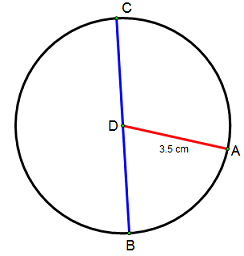# How to Find the Diameter of a Circle: Definition, Formula & Example

Lesson Transcript
Instructor: Miriam Snare

Miriam has taught middle- and high-school math for over 10 years and has a master's degree in Curriculum and Instruction.

To find the diameter of a circle, multiply the circle's radius by two. Learn the definition of a diameter and a radius and review a detailed explanation of the formula used to find the diameter of a circle with examples. Updated: 10/11/2021

## Definition

The diameter of a circle is a segment whose endpoints lie on the circle and whose midpoint is the center of the circle. When someone says 'lie on the circle,' that means on the outline that traces the circle, not the space within that outline. The center of the circle is a point exactly in the middle of the space within the outline. The center is also the same distance away from every point on the outline of the circle. The distance from the center to a point on the circle is called a radius.An error occurred trying to load this video.

Try refreshing the page, or contact customer support.

Coming up next: Math Grids: Examples, Overview

### You're on a roll. Keep up the good work!

Replay
Your next lesson will play in 10 seconds
• 0:00 Definition
• 0:40 Examples
• 1:37 Formula
• 2:39 Lesson Summary
Save Save

Want to watch this again later?

Timeline
Autoplay
Autoplay
Speed Speed

## Examples

The segment AB is a diameter. Point C is the center of the circle, and it is also the midpoint of segment AB. Segments AC and CB are of equal length and are each half the length of the diameter. AC and CB are each a radius of the circle. A radius of a circle is a segment with one endpoint on the circle and the other endpoint at the center of the circle.There is only one diameter drawn on circle C. However, every circle has an infinite number of possible diameters. Imagine you have to cut a round cookie into two equal pieces. No matter how you turn the cookie, as long as you make one straight cut right through the center point of the cookie, you will split it along a diameter.

## Formula

The formula to find the diameter states the relationship between the diameter and the radius. The diameter is made up of two segments that are each a radius. Therefore, the formula is: Diameter = 2 * the measurement of the radius. You can abbreviate this formula as d=2r.To unlock this lesson you must be a Study.com Member.

### Register to view this lesson

Are you a student or a teacher?